# If Cell is Not Blank

IF function is frequently used in Excel worksheet to return you expect “true value” or “false value” based on the result of created logical test. If you want to see if a cell is blank or not, and leave some comments for not blank cells, you can build a formula with IF function, IF function can return “value if not blank” or “value if blank” based on test “if cell is NOT BLANK”. See example below.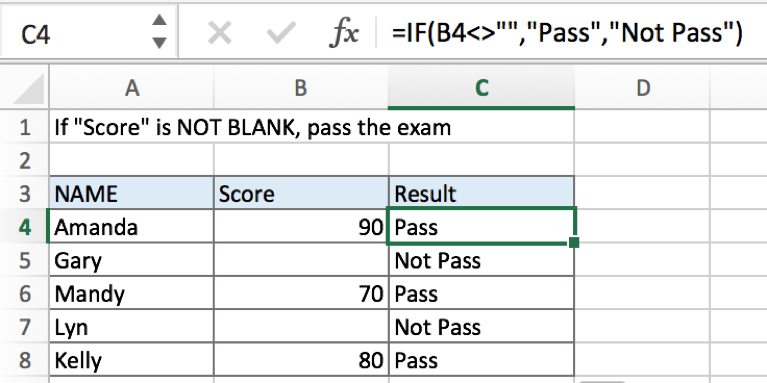In above example, if cell is not blank in B column for a student, we think he passed the exam, formula returns result of true “Pass”, otherwise for a student, if there is no score in B column, we can assume that he didn’t pass the exam, so formula retrieves false value from the two results and returns “Not Pass” for him.

## FORMULA

To test if cell equals a certain text string, the generic formula is:

=IF(A1<>””,”if not blank value”,”blank value”)

Formula in this example:

=IF(B4<>””,”Pass”,”Not Pass”)

## EXPLANATION

In this example, for a student, if cell in “Score” column is not blank, that means he passed the exam, in this case, we want to fill “Pass” in “Result” column. On the other side, if cell is blank, we want to fill “Not pass” for those students who failed the exam. So, there are two results by design, pick which one from the two result is based on the result of the logical test “if cell is blank or not”, in Excel, IF function can handle this case effectively.

IF function allows you to create a logical comparison between your value and reference value (for example “A1>0”), and set true value and false value what you expect to return as test results. IF function returns one of the two results based on logical comparison result.

Syntax: IF(logica_test,[value_if_true],[value_if_false])

To test if B4 is not blank, we can directly create a logical comparison B4<>””. The symbol <> is a logical operator that means “not equal to”. And double quotes “” represents “Empty”, there is no need to add an extra space between “”, but double quotes “” should not be ignored. If missing double quotes, Excel will prompts warning message that the formula you typed contains an error, if you ignore the error, #VALUE! is displayed in cell. Above all, B4<>”” means B4 is not equal to empty.

Besides, for the two arguments “value_if_true” and “value_if_false”, we set “Pass” and “Not Pass” separately. So the formula is: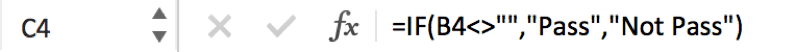In this example, B4 is not a blank cell, logical test B4<>”” is true, so IF function evaluates True value “Pass” to C4.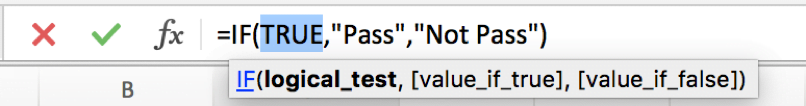For B5, B5 is a blank cell, B5<>”” is false, so IF retrieves False value “Not Pass” to C5.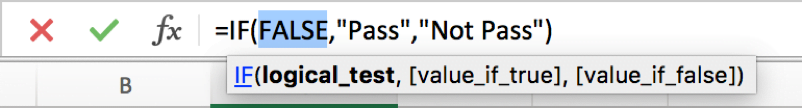In IF, true value and false value can be set as empty string “”, a text, a number or a formula, you can set what you expect to these two values.

## ISBLANK Function

You can also use ISBLANK function to test if a cell is blank or not. In the formula, we can use ISBLANK(B4) to replace original logical test B4<>””. But we should be aware that ISBLANK function is to test if a cell is blank, so it returns true value if it detects that a cell is blank. So, the two results of true and false should be reversed.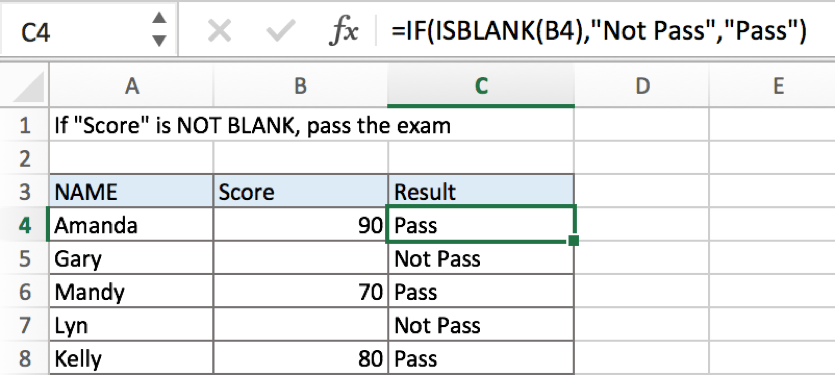If you want to keep the sequence of your two results, you can add NOT function before ISBLANK.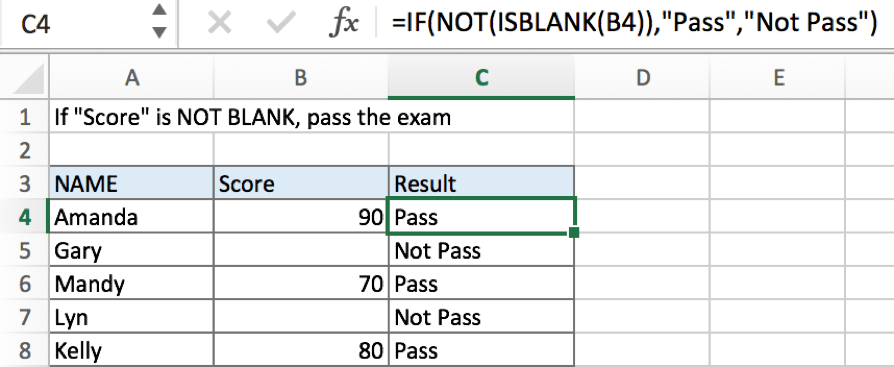### Related Functions

• Excel ISBLANK function
The Excel ISBLANK function returns TRUE if the value is blank or null.The syntax of the ISBLANK function is as below:= ISBLANK (value)…
• Excel IF function
The Excel IF function perform a logical test to return one value if the condition is TRUE and return another value if the condition is FALSE. The IF function is a build-in function in Microsoft Excel and it is categorized as a Logical Function.The syntax of the IF function is as below:= IF (condition, [true_value], [false_value])….